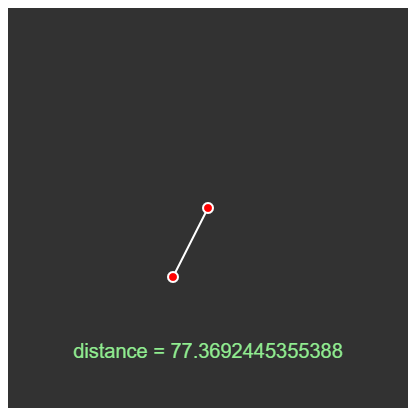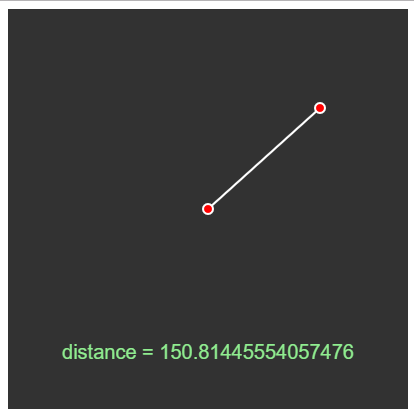# p5.js | dist() Function

The dist() function calculates the Euclidean distance in 2D or 3D. Means the p5.js | dist() Function is used to measure the distance between two points in 2D or 3D. Below is the formula for distance in 2D and 3D.

• 2D formula:• 3D formula:Syntax:

dist(x1, y1, x2, y2)

dist(x1, y1, z1, x2, y2, z2)


Parameters:

• x1: These parameters holds the x-coordinate of the first point.
• y1: These parameters hold the y-coordinate of the first point.
• z1: These parameters hold the z-coordinate of the first point.
• x2: These parameters hold the x-coordinate of the second point.
• y2: These parameters hold the y-coordinate of the second point.
• z2: These parameters hold the z-coordinate of the second point.

Return value: Distance between two points.
Example: This example calculates and prints the distance between a fixed point and the cursor.

 function setup() {      // Create a canvas      createCanvas(400, 400);  }     function draw() {      // set background color      background(50);      // coordinates of the fixed point      var x1 = 200;      var y1 = 200;      // coordinates of the cursor      var x2 = mouseX;      var y2 = mouseY;      // set line color and weight      stroke(255);      strokeWeight(2);      // draw a line connecting 2 points      line(x1, y1, x2, y2);      fill("red");      // draw a circle centered at each point      ellipse(x1, y1, 10);      ellipse(x2, y2, 10);      // calculate the distance between 2 points      d = dist(x1, y1, x2, y2);      noStroke();      fill("lightgreen");      // set text size and alignment      textSize(20);      textAlign(CENTER);      // display the distance calculated      text("distance = "+ str(d), 200, 350);  }

Output:Online editor: https://editor.p5js.org/
Environment Setup: https://www.geeksforgeeks.org/p5-js-soundfile-object-installation-and-methods/

Reference: https://p5js.org/reference/#/p5/dist

My Personal Notes arrow_drop_upCheck out this Author's contributed articles.

If you like GeeksforGeeks and would like to contribute, you can also write an article using contribute.geeksforgeeks.org or mail your article to contribute@geeksforgeeks.org. See your article appearing on the GeeksforGeeks main page and help other Geeks.

Please Improve this article if you find anything incorrect by clicking on the "Improve Article" button below.

Improved By : ManasChhabra2

Article Tags :

Be the First to upvote.

Please write to us at contribute@geeksforgeeks.org to report any issue with the above content.